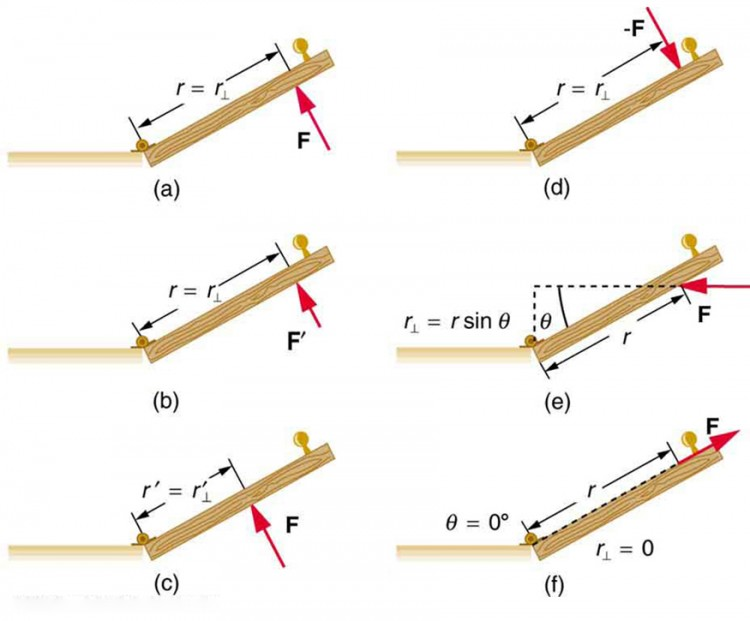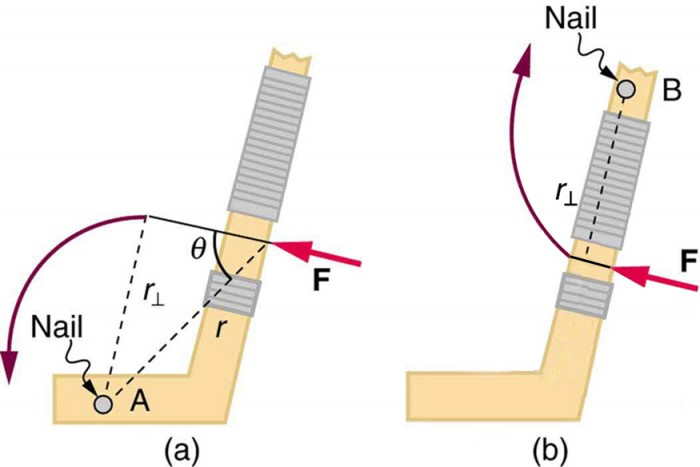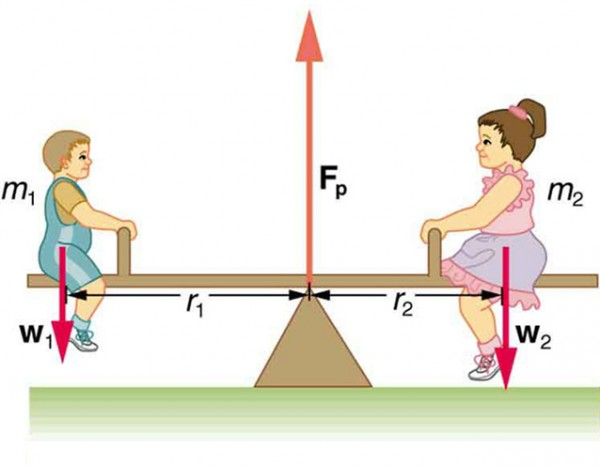The Second Condition for Equilibrium

Learning Objectives

By the end of this section, you will be able to:

• State the second condition that is necessary to achieve equilibrium.
• Explain torque and the factors on which it depends.
• Describe the role of torque in rotational mechanics.

Torque

The second condition necessary to achieve equilibrium involves avoiding accelerated rotation (maintaining a constant angular velocity. A rotating body or system can be in equilibrium if its rate of rotation is constant and remains unchanged by the forces acting on it. To understand what factors affect rotation, let us think about what happens when you open an ordinary door by rotating it on its hinges.
Several familiar factors determine how effective you are in opening the door. See Figure 1. First of all, the larger the force, the more effective it is in opening the door—obviously, the harder you push, the more rapidly the door opens. Also, the point at which you push is crucial. If you apply your force too close to the hinges, the door will open slowly, if at all. Most people have been embarrassed by making this mistake and bumping up against a door when it did not open as quickly as expected. Finally, the direction in which you push is also important. The most effective direction is perpendicular to the door—we push in this direction almost instinctively.Figure 1. Torque is the turning or twisting effectiveness of a force, illustrated here for door rotation on its hinges (as viewed from overhead). Torque has both magnitude and direction. (a) Counterclockwise torque is produced by this force, which means that the door will rotate in a counterclockwise due to F. Note that r is the perpendicular distance of the pivot from the line of action of the force. (b) A smaller counterclockwise torque is produced by a smaller force F′ acting at the same distance from the hinges (the pivot point). (c) The same force as in (a) produces a smaller counterclockwise torque when applied at a smaller distance from the hinges. (d) The same force as in (a), but acting in the opposite direction, produces a clockwise torque. (e) A smaller counterclockwise torque is produced by the same magnitude force acting at the same point but in a different direction. Here, θ is less than 90º. (f) Torque is zero here since the force just pulls on the hinges, producing no rotation. In this case, θ = 0º.

The magnitude, direction, and point of application of the force are incorporated into the definition of the physical quantity called torque. Torque is the rotational equivalent of a force. It is a measure of the effectiveness of a force in changing or accelerating a rotation (changing the angular velocity over a period of time). In equation form, the magnitude of torque is defined to be

$\tau = rF \sin \theta\\$

where τ (the Greek letter tau) is the symbol for torque, r is the distance from the pivot point to the point where the force is applied, F is the magnitude of the force, and θ is the angle between the force and the vector directed from the point of application to the pivot point, as seen in Figure 1 and Figure 2. An alternative expression for torque is given in terms of the perpendicular lever arm r as shown in Figure 1 and Figure 2, which is defined as

r⊥ = r sin θ

so that

τ rF.Figure 2. A force applied to an object can produce a torque, which depends on the location of the pivot point. (a) The three factors r, F, and θ for pivot point A on a body are shown here—r is the distance from the chosen pivot point to the point where the force F is applied, and θ is the angle between F and the vector directed from the point of application to the pivot point. If the object can rotate around point A, it will rotate counterclockwise. This means that torque is counterclockwise relative to pivot A. (b) In this case, point B is the pivot point. The torque from the applied force will cause a clockwise rotation around point B, and so it is a clockwise torque relative to B.

The perpendicular lever arm r is the shortest distance from the pivot point to the line along which F acts; it is shown as a dashed line in Figure 1 and Figure 2. Note that the line segment that defines the distance r is perpendicular to F, as its name implies. It is sometimes easier to find or visualize r than to find both r and θ. In such cases, it may be more convenient to use τ rF rather than τ = rF sin θ for torque, but both are equally valid.

The SI unit of torque is newtons times meters, usually written as N · m. For example, if you push perpendicular to the door with a force of 40 N at a distance of 0.800 m from the hinges, you exert a torque of 32 N · m (0.800 m × 40 N × sin 90º) relative to the hinges. If you reduce the force to 20 N, the torque is reduced to 16 N · m, and so on.

The torque is always calculated with reference to some chosen pivot point. For the same applied force, a different choice for the location of the pivot will give you a different value for the torque, since both r and θ depend on the location of the pivot. Any point in any object can be chosen to calculate the torque about that point. The object may not actually pivot about the chosen “pivot point.”

Note that for rotation in a plane, torque has two possible directions. Torque is either clockwise or counterclockwise relative to the chosen pivot point, as illustrated for points B and A, respectively, in Figure 2. If the object can rotate about point A, it will rotate counterclockwise, which means that the torque for the force is shown as counterclockwise relative to A. But if the object can rotate about point B, it will rotate clockwise, which means the torque for the force shown is clockwise relative to B. Also, the magnitude of the torque is greater when the lever arm is longer.

Now, the second condition necessary to achieve equilibrium is that the net external torque on a system must be zero. An external torque is one that is created by an external force. You can choose the point around which the torque is calculated. The point can be the physical pivot point of a system or any other point in space—but it must be the same point for all torques. If the second condition (net external torque on a system is zero) is satisfied for one choice of pivot point, it will also hold true for any other choice of pivot point in or out of the system of interest. (This is true only in an inertial frame of reference.) The second condition necessary to achieve equilibrium is stated in equation form as

net τ = 0

where net means total. Torques, which are in opposite directions are assigned opposite signs. A common convention is to call counterclockwise (ccw) torques positive and clockwise (cw) torques negative. When two children balance a seesaw as shown in Figure 3, they satisfy the two conditions for equilibrium. Most people have perfect intuition about seesaws, knowing that the lighter child must sit farther from the pivot and that a heavier child can keep a lighter one off the ground indefinitely.Figure 3. Two children balancing a seesaw satisfy both conditions for equilibrium. The lighter child sits farther from the pivot to create a torque equal in magnitude to that of the heavier child.

Example 1. She Saw Torques On A Seesaw

The two children shown in Figure 3 are balanced on a seesaw of negligible mass. (This assumption is made to keep the example simple—more involved examples will follow.) The first child has a mass of 26.0 kg and sits 1.60 m from the pivot.(a) If the second child has a mass of 32.0 kg, how far is she from the pivot? (b) What is Fp, the supporting force exerted by the pivot?

Strategy

Both conditions for equilibrium must be satisfied. In part (a), we are asked for a distance; thus, the second condition (regarding torques) must be used, since the first (regarding only forces) has no distances in it. To apply the second condition for equilibrium, we first identify the system of interest to be the seesaw plus the two children. We take the supporting pivot to be the point about which the torques are calculated. We then identify all external forces acting on the system.

Solution (a)

The three external forces acting on the system are the weights of the two children and the supporting force of the pivot. Let us examine the torque produced by each. Torque is defined to be

τ = rF sin θ

Here θ = 90º, so that sin θ = 1  for all three forces. That means r⊥ r for all three. The torques exerted by the three forces are first,

τ1 = r 1 w1

second,

τ2 = – r2w2

and third,

$\begin{array}{lll}{\tau }_{\text{p}}& =& {r}_{\text{p}}{F}_{\text{p}}\\ & =& 0\cdot {F}_{\text{p}}\\ & =& 0.\end{array}\\$

Note that a minus sign has been inserted into the second equation because this torque is clockwise and is therefore negative by convention. Since Fp acts directly on the pivot point, the distance rp is zero. A force acting on the pivot cannot cause a rotation, just as pushing directly on the hinges of a door will not cause it to rotate. Now, the second condition for equilibrium is that the sum of the torques on both children is zero. Therefore

τ= –τ1,

or

r2wr1w1.

Weight is mass times the acceleration due to gravity. Entering mg for w, we get

r2m2r1m1g.

Solve this for the unknown r2:

${r}_{2}={r}_{1}\frac{{m}_{1}}{{m}_{2}}\\$

The quantities on the right side of the equation are known; thus, r2 is

${r}_{2}=\left(\text{1.60 m}\right)\frac{\text{26.0 kg}}{\text{32.0 kg}}=\text{1.30 m}\\$

As expected, the heavier child must sit closer to the pivot (1.30 m versus 1.60 m) to balance the seesaw.

Solution (b)

This part asks for a force Fp. The easiest way to find it is to use the first condition for equilibrium, which is

net F = 0

The forces are all vertical, so that we are dealing with a one-dimensional problem along the vertical axis; hence, the condition can be written as

net Fy = 0

where we again call the vertical axis the y-axis. Choosing upward to be the positive direction, and using plus and minus signs to indicate the directions of the forces, we see that

Fpw1w= 0.

This equation yields what might have been guessed at the beginning:

Fw1+w2.

So, the pivot supplies a supporting force equal to the total weight of the system:

Fm1m2g.

Entering known values gives

$\begin{array}{lll}{F}_{\text{p}}& =& \left(\text{26.0 kg}\right)\left(9.80{\text{m/s}}^{2}\right)+\left(\text{32.0 kg}\right)\left(9.80{\text{m/s}}^{2}\right)\\ & =& \text{568 N.}\end{array}\\$

Discussion

The two results make intuitive sense. The heavier child sits closer to the pivot. The pivot supports the weight of the two children. Part (b) can also be solved using the second condition for equilibrium, since both distances are known, but only if the pivot point is chosen to be somewhere other than the location of the seesaw’s actual pivot!

Several aspects of the preceding example have broad implications. First, the choice of the pivot as the point around which torques are calculated simplified the problem. Since Fp is exerted on the pivot point, its lever arm is zero. Hence, the torque exerted by the supporting force Fp is zero relative to that pivot point. The second condition for equilibrium holds for any choice of pivot point, and so we choose the pivot point to simplify the solution of the problem.

Second, the acceleration due to gravity canceled in this problem, and we were left with a ratio of masses. This will not always be the case. Always enter the correct forces—do not jump ahead to enter some ratio of masses.

Third, the weight of each child is distributed over an area of the seesaw, yet we treated the weights as if each force were exerted at a single point. This is not an approximation—the distances r1 and r2 are the distances to points directly below the center of gravity of each child. As we shall see in the next section, the mass and weight of a system can act as if they are located at a single point.

Finally, note that the concept of torque has an importance beyond static equilibrium. Torque plays the same role in rotational motion that force plays in linear motion. We will examine this in the next chapter.

Take-Home Experiment

Take a piece of modeling clay and put it on a table, then mash a cylinder down into it so that a ruler can balance on the round side of the cylinder while everything remains still. Put a penny 8 cm away from the pivot. Where would you need to put two pennies to balance? Three pennies?

Section Summary

• The second condition assures those torques are also balanced. Torque is the rotational equivalent of a force in producing a rotation and is defined to be
$\tau = rF \sin \theta\\$

where τ is torque, r, is the distance from the pivot point to the point where the force is applied, F is the magnitude of the force, and θ is the angle between F and the vector directed from the point where the force acts to the pivot point. The perpendicular lever arm r is defined to be

${r}_{\perp }=r\sin{\theta}\\$

so that

$\tau ={r}_{\perp }F\\$.
• The perpendicular lever arm r is the shortest distance from the pivot point to the line along which F acts. The SI unit for torque is newton-meter N · m. The second condition necessary to achieve equilibrium is that the net external torque on a system must be zero:
• $\text{net}\tau =0\\$

By convention, counterclockwise torques are positive, and clockwise torques are negative.

Conceptual Questions

1. What three factors affect the torque created by a force relative to a specific pivot point?

2. A wrecking ball is being used to knock down a building. One tall unsupported concrete wall remains standing. If the wrecking ball hits the wall near the top, is the wall more likely to fall over by rotating at its base or by falling straight down? Explain your answer. How is it most likely to fall if it is struck with the same force at its base? Note that this depends on how firmly the wall is attached at its base.

3. Mechanics sometimes put a length of pipe over the handle of a wrench when trying to remove a very tight bolt. How does this help? (It is also hazardous since it can break the bolt.)

Problems & Exercises

1. (a) When opening a door, you push on it perpendicularly with a force of 55.0 N at a distance of 0.850m from the hinges. What torque are you exerting relative to the hinges? (b) Does it matter if you push at the same height as the hinges?

2. When tightening a bolt, you push perpendicularly on a wrench with a force of 165 N at a distance of 0.140 m from the center of the bolt. (a) How much torque are you exerting in newton × meters (relative to the center of the bolt)? (b) Convert this torque to footpounds.

3. Two children push on opposite sides of a door during play. Both push horizontally and perpendicular to the door. One child pushes with a force of 17.5 N at a distance of 0.600 m from the hinges, and the second child pushes at a distance of 0.450 m. What force must the second child exert to keep the door from moving? Assume friction is negligible.

4. Use the second condition for equilibrium net τ = 0 to calculate Fp in Example 1, employing any data given or solved for in part (a) of the example.

5. Repeat the seesaw problem in Example 1 with the center of mass of the seesaw 0.160 m to the left of the pivot (on the side of the lighter child) and assuming a mass of 12.0 kg for the seesaw. The other data given in the example remain unchanged. Explicitly show how you follow the steps in the Problem-Solving Strategy for static equilibrium.

Glossary

torque:
turning or twisting effectiveness of a force
perpendicular lever arm:
the shortest distance from the pivot point to the line along which F lies
SI units of torque:
newton times meters, usually written as N·m
center of gravity:
the point where the total weight of the body is assumed to be concentrated

Selected Solutions to Problems & Exercises

1. (a) 46.8 N·m (b) It does not matter at what height you push. The torque depends on only the magnitude of the force applied and the perpendicular distance of the force’s application from the hinges. (Children don’t have a tougher time opening a door because they push lower than adults, they have a tougher time because they don’t push far enough from the hinges.)

3. 23.3 N

5. Given:

$\begin{array}{ccc}{m}_{1}& =& \text{26.0 kg,}{m}_{2}=\text{32.0 kg,}{m}_{\text{s}}=\text{12.0 kg,}\\ {r}_{1}& =& \text{1.60 m,}{r}_{\text{s}}=\text{0.160 m, find (a)}{r}_{2,}\text{(b)}{F}_{\text{p}}\end{array}\\$

a) Since children are balancing:

$\begin{array}{c}\text{net }{\tau}_{\text{cw}}=-\text{net }{\tau }_{\text{ccw}}\\ \Rightarrow{w}_{1}{r}_{1}+{m}_{\text{s}}{gr}_{\text{s}}={w}_{2}{r}_{2}\\ \end{array}\\$

So, solving for r2 gives:

$\begin{array}{lll}{r}_{2}& =& \frac{{w}_{1}{r}_{1}+{m}_{\text{s}}{\mathit{gr}}_{\text{s}}}{{w}_{2}}=\frac{{m}_{1}{\mathit{gr}}_{1}+{m}_{\text{s}}{\mathit{gr}}_{\text{s}}}{{m}_{2}g}=\frac{{m}_{1}{r}_{1}+{m}_{\text{s}}{r}_{\text{s}}}{{m}_{2}}\\ & =& \frac{\left(\text{26.0 kg}\right)\left(\text{1.60 m}\right)+\left(\text{12.0 kg}\right)\left(\text{0.160 m}\right)}{\text{32.0 kg}}\\ & =& \text{1.36 m}\end{array}\\$

b) Since the children are not moving:

$\begin{array}{c}\text{net } F=0={{F}_{\text{p}}}-{{w}_{1}}-{{w}_{2}}-{{w}_{\text{s}}}\\ \Rightarrow {F}_{\text{p}}={{w}_{1}}+{{w}_{2}}+{{w}_{\text{s}}}\end{array}\\$

So that

$\begin{array}{lll}{F}_{\text{p}}& =& \left(\text{26.0 kg}+\text{32.0 kg}+\text{12.0 kg}\right)\left(\text{9.80}\text{m}/{\text{s}}^{2}\right)\\ & =& \text{686 N}\end{array}\\$